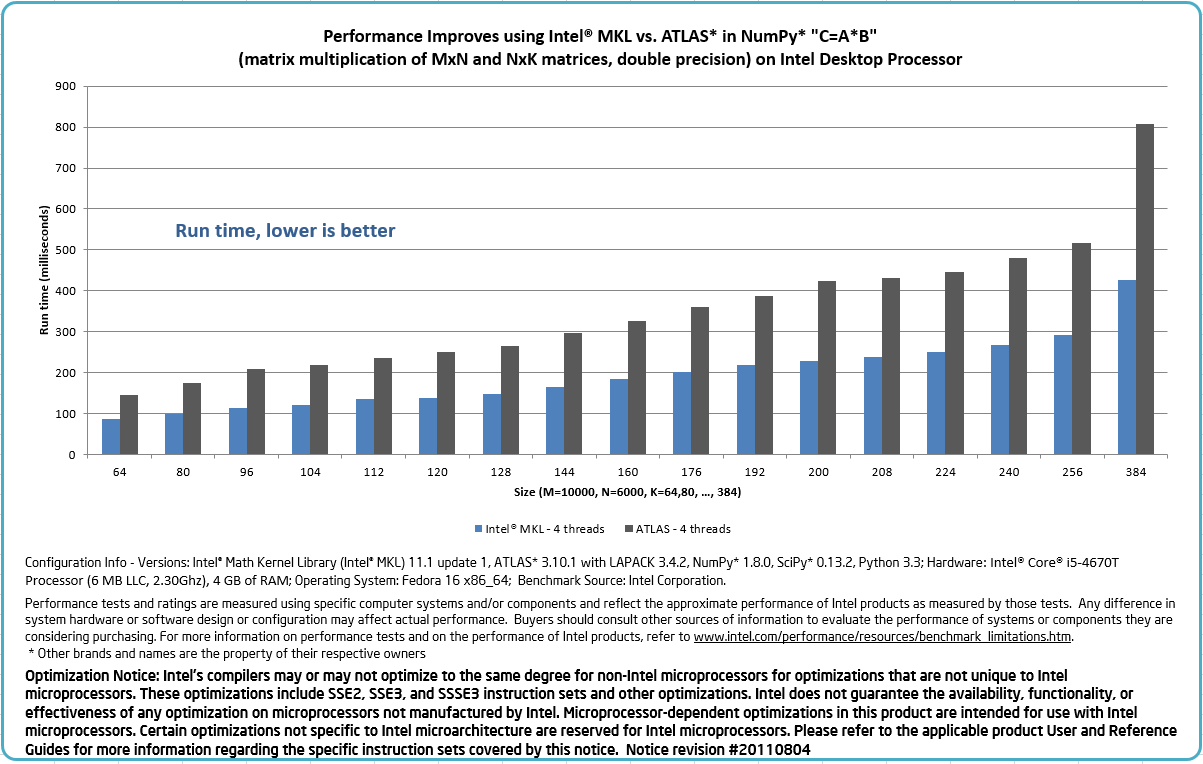# [AI]关于SkLearn 及其 性能问题

2019/01/08 09:39

## Scikit-Learn 使用

Scikit-Learn (sklearn) 把很多学习模型抽象好 'NB', 'KNN', 'LR', 'RF', 'DT', 'SVM', 'GBDT'，可以通过统一接口返回model对象，然后对它进行fit,predict等操作。

Scikit-Learn Sample

# Multinomial Naive Bayes Classifier
def naive_bayes_classifier(train_x, train_y):
from sklearn.naive_bayes import MultinomialNB
model = MultinomialNB(alpha=0.01)
model.fit(train_x, train_y)
return model

# KNN Classifier
def knn_classifier(train_x, train_y):
from sklearn.neighbors import KNeighborsClassifier
model = KNeighborsClassifier()
model.fit(train_x, train_y)
return model

# Logistic Regression Classifier
def logistic_regression_classifier(train_x, train_y):
from sklearn.linear_model import LogisticRegression
model = LogisticRegression(penalty='l2')
model.fit(train_x, train_y)
return model

# 其他模型也是类似方式： 'NB', 'KNN', 'LR', 'RF', 'DT', 'SVM', 'GBDT'



predict = model.predict(test_x)
accuracy = metrics.accuracy_score(test_y, predict)


## sklearn 性能问题

### GIL (Global Interpreter Lock 全局解释器锁)

GIL 与 Python 线程的纠葛

### MKL（Intel® Math Kernel Library)

Numpy/Scipy可以使用MKL库提升计算性能Numpy+MKL不能使用pip3直接安装，可以在下面的地址下载离线安装包。 Numpy+MKL安装地址0
0 收藏

### 作者的其它热门文章0 评论
0 收藏
0